Importance: High ✭✭✭
 Author(s): Behzad, M.
 Subject: Graph Theory » Coloring
 Keywords: Total coloring
 Recomm. for undergrads: no
 Posted by: Iradmusa on: June 4th, 2008
Conjecture   A total coloring of a graph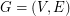is an assignment of colors to the vertices and the edges ofsuch that every pair of adjacent vertices, every pair of adjacent edges and every vertex and incident edge pair, receive different colors. The total chromatic number of a graph,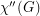, equals the minimum number of colors needed in a total coloring of. It is an old conjecture of Behzad that for every graph, the total chromatic number equals the maximum degree of a vertex in,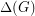plus one or two. In other words,The lower bound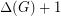is trivial by looking at the number of colours required on a vertex of maximum degree and its incident edges. It is easy to prove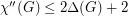. Molloy and Reed [MR] showed that there exists a constantsuch that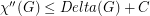for every graph.

The Edge list coloring conjecture would imply that.

The Total Colouring Conjecture was proved forby Rosenfeld [R] and also by Vijayaditya [V], and for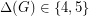by Kostochka [K1,K2,K3]; in fact the proof for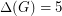holds for multigraphs.

The Conjecture has also been established for many graph classes. For every planar graph G with, the following clever argument proves it. By the 4 Color Theorem, we can color the vertices with the colors 1, 2, 3, 4. By a result of Sanders and Zhao [SZ], we can color the edges of the graph with the colors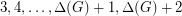. Uncolor each edge that was colored 3 or 4. Note that each uncolored edge has exactly two colors fromforbidden. Hence, each uncolored edge has at least two colors available. Note that the uncolored edges induce a disjoint union of paths and even cycles. Thus, by a special case of a theorem of Erdos, Rubin, and Taylor [ERT], we can color the edges from their lists of two available colors each.

## Bibliography

*[B] M. Behzad, Graphs and their chromatic numbers, Ph.D. Thesis, Michigan State University, 1965.

[ERT] P. Erdos, A.L. Rubin, and H. Taylor, Choosability in graph, Cong. Numer. 26, 125-157, 1979.

[K1] A.V. Kostochka, The total coloring of a multigraph with maximal degree 4. Discrete Math. 17, 161-163, 1977.

[K2] A.V. Kostochka, Upper bounds of chromatic functions of graph (in Russian). Ph.D. Thesis, Novosibirsk, 1978.

[K3] A.V. Kostochka, Exact upper bound for the total chromatic number of a graph (in Russian). In: Proc. 24th Int. Wiss. Koll., Tech Hochsch. Ilmenau, 1979, pages 33-36, 1979.

[MR] M. Molloy and B.Reed. A bound on the total chromatic number. Combinatorica, 18(2), 241-280, 1998.

[R] M. Rosenfeld, On the total coloring of certain graphs. Israel J. Math. 9, 396-402, 1971.

[SZ] D.P. Sanders and Y. Zhao, Planar Graphs of Maximum Degree Seven are Class 1. J. Comb. Theory B. 83, 201-212, 2001.

[V] N. Vijayaditya, On total chormatic number of a graph. J. London Math. Soc. (2) 3, 405-408, 1971.

* indicates original appearance(s) of problem.

## Reply

More information about formatting options